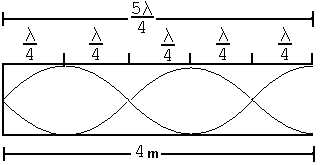# Phy 121 Ch 13-15 Exam Name

 Page 1/2 Date 20.05.2018 Size 96.38 Kb. #49552
1   2
PHY 121 Ch 13-15 Exam Name

 When a 200 gr mass is attached to a vertical spring, it causes the spring to stretch a distance d. OR ω 2 = k / m (2πf)2 = k / 0.2 f = 100 osc / 50 sec (2π2)2 = k / 0.2 k = 31.5 N/m 15/15 -k d = .2 g 31.6(d) = .2(9.8) d = 0.0621 m If the mass is now displaced slightly from equilibrium, it is found to make 100 oscillations in 50.0 s. What is d ? 10/10 7/7 k = 4 π2 m / T2 k = 4 π2 .2/(50/100)2 k = 31.6 N/m

 An organ pipe 4.00m long is open at one end, similar to your lab. What is the linear distance between a node and the adjacent antinode for the 5th harmonic in this organ pipe? (Hint, this is easier if you draw in the nodes/antinodes) 5λ/4 = 4 m  10/10 λ = 16/5 meters N to A is λ / 4  10/10 N to A = 16/5 / 4 = 4/5 meters 12/12Through a pipe 15.0 cm in diameter, water is pumped from the Colorado River up to Grand Canyon Village, located on the rim of the canyon. The river is at an elevation of 564 m and the village is at an elevation of 2096 m. What is the pressure to deliver a flow of 4500 m3 per day? 4/4 8/8 4500 m3/d (1d/24h)(24h/3600s) = A v 0.052083 = π¼d2 v 0.052083 = π¼ .152 v v = 2.95 m/s 4/4 4/4 8/8 8/8 P + ½ρv2 + ρgh = P2 + ½ ρ v22 + ρ g h2 (1atm + Ppump) + 0 + 0 = 1atm + ½ 1000 2.952 + 1000(9.8)(2096-564) Ppump = 4340 Pa + 150 x 105 Pa Ppump = 150.043 x 105 Pa (The level of the river doesn’t lower appreciably so v is about zero)